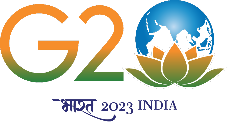Faculties
List of Faculties in Mathematics Department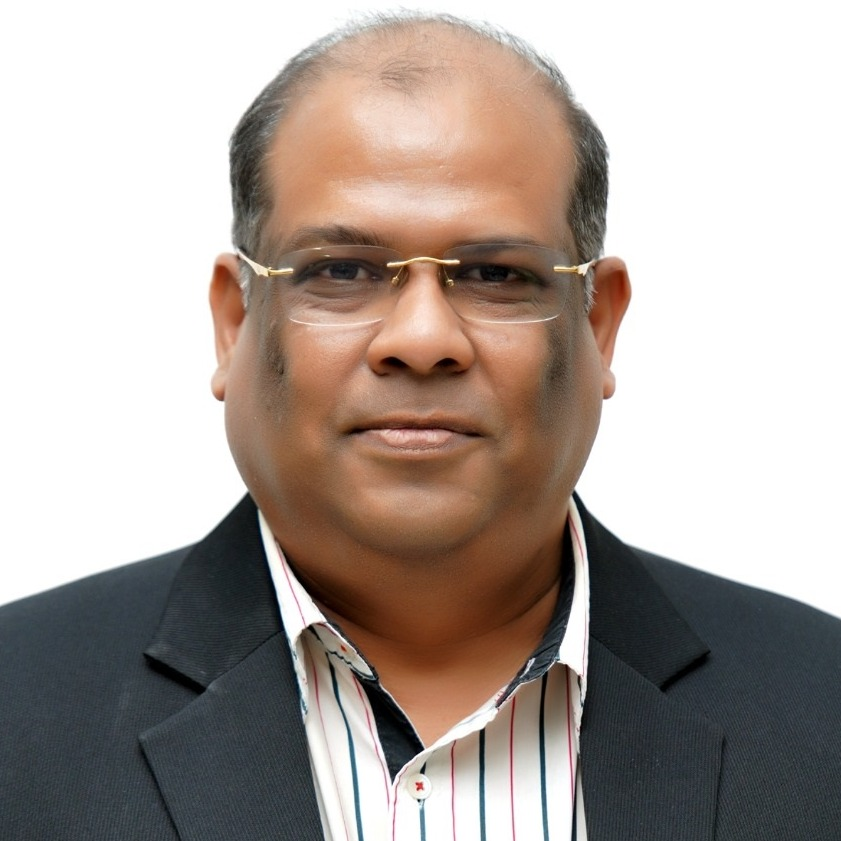Sandip Banerjee
Professor
sandip.banerjee[at]ma.iitr.ac.in
(01332)285697
Mathematical Biology, Mathematical Modeling.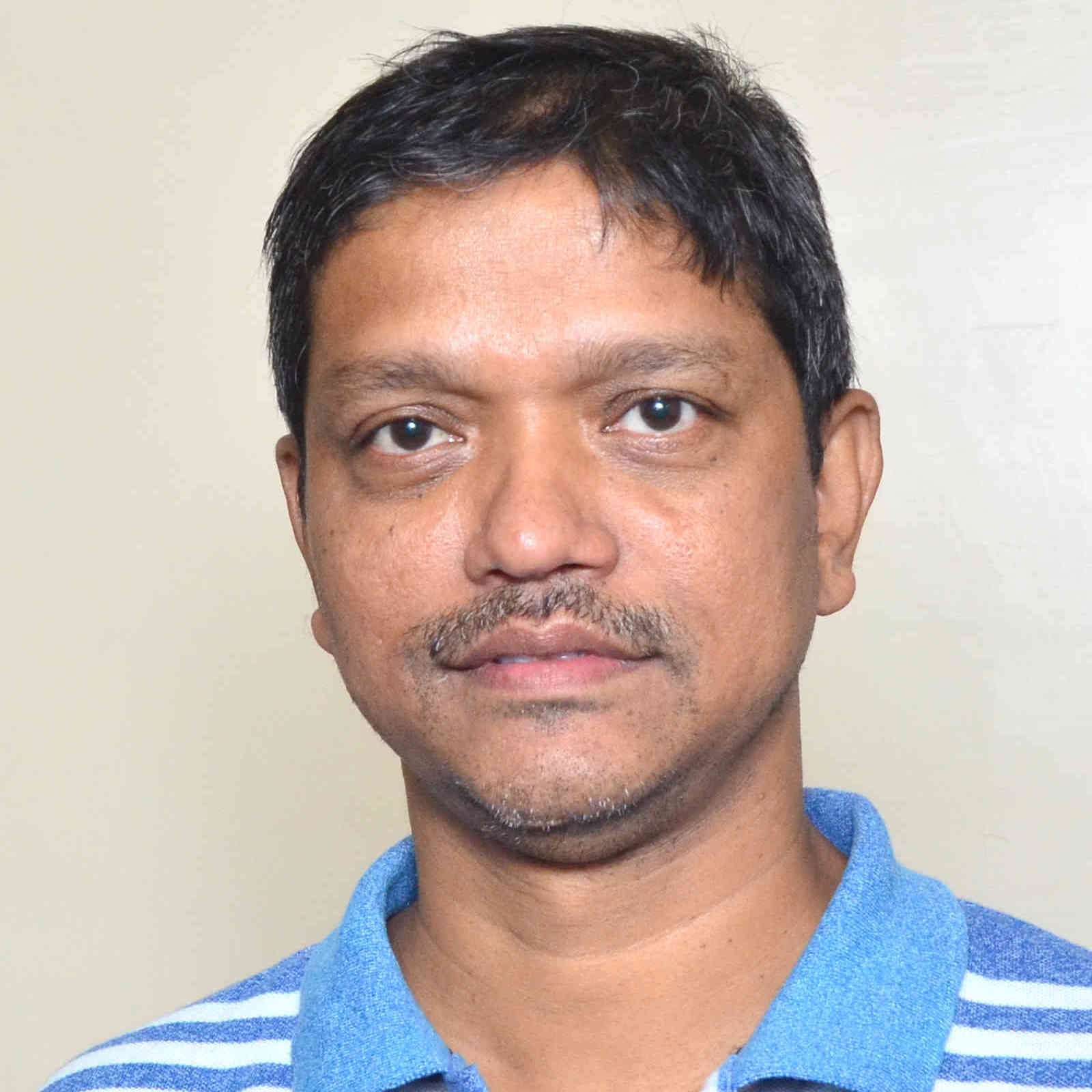Premananda Bera
p.bera[at]ma.iitr.ac.in
01332-285071
Hydrodynamic stability in multiphase flow, Hydrodynamics using Spectral methods / Spectral element methods / finite difference methods,, Convection in porous media, Non-conforming spectral element methods: theory and applications, Mechanisms of miscible fluid flow in porous medium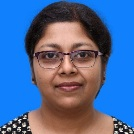Associate professor
01332-285829
Statistics and related areas, Data analytics, Discrete Probability, Cryptography, Financial Mathematics, Statistical Inference.Surojit Ghosh
Assistant professor
surojit.ghosh[at]ma.iitr.ac.in
8981082047
Algebraic Topology, Equivariant homotopy theoryAnkik Kumar Giri
Associate professor
ankik.giri[at]ma.iitr.ac.in
Partial Integro-Differential Equations, Coagulation-Fragmenation Models: Well-posedness, Regularity, Mass-conservation, Gelation, Large time behaviour, Stationary solutions, Self-similar profiles, Numerical Methods, Error Analysis, Stochastic Partial Differential Equations: Well-posedness, Regularity and Convergence Analysis of Numerical Methods, Regularization Theory for Inverse Problems: Variational Regularization and Iterative regularization, Stability Estimates, Regularized Solutions, Convergence, Convergence Rates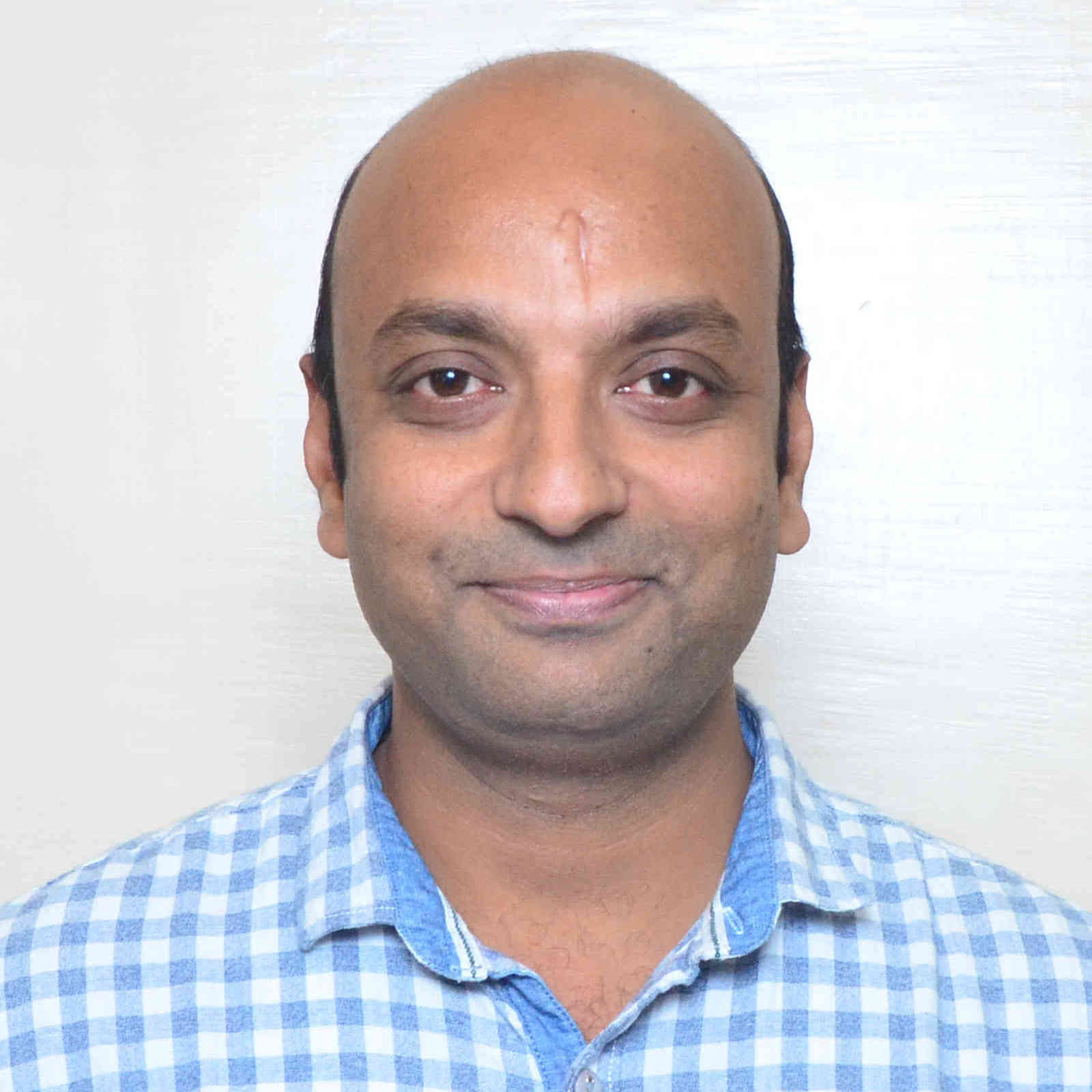Shiv Kumar Gupta
Associate professor
s.gupta[at]ma.iitr.ac.in
+91-1332-285379
Optimization theory, Duality, Support Vector Machines, Fuzzy mathematical programming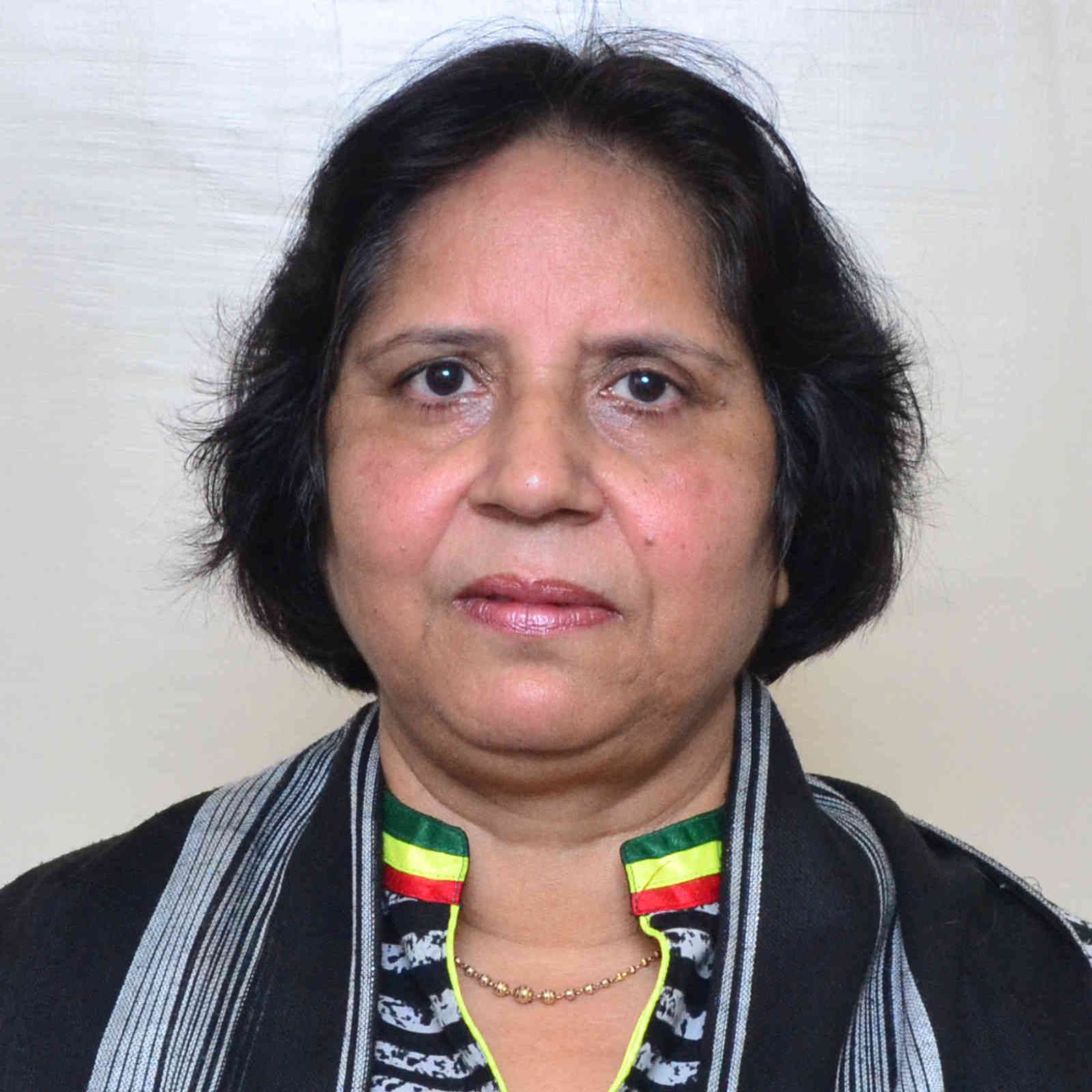Associate professor
08126379111
Computer Communications Networks, Performance Prediction of Wireless Systems, Networking, , Operations Research, Stochastic Processes, Queueing theory, Inventory Models, Software and hardware , Industrial Mathematics, Mathematical Modelling, BiomathematicsRam Jiwari
Associate professor
ram.jiwari[at]ma.iitr.ac.in
8191957249
Numerical Analysis, Numerical Analysis and Simulations of PDEs, Wavelets Analysis, Finite Element Methods, Computational Modeling, Lie Symmetry AnalysisArbaz Khan
Assistant professor
arbaz[at]ma.iitr.ac.in
+91-1332-284891
Numerical Solution of Partial Differential Equations, h-p finite element/Spectral Element Methods, Least Squares Method, Discontinuous Galerkin (DG) FEM, HDG FEM, A Posteriori Error Analysis, Multigrid Method, Parallel Computing, Domain Decomposition, Scientific Computing, Mixed FEM, Eigenvalue problems, Numerical Linear Algebra, Uncertainty Quantification, Stochastic Partial Differential Equation, Adaptivity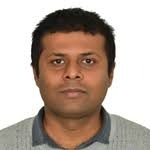Chaman Kumar
Assistant professor
c.kumarfma[at]iitr.ac.in
M 109, Department of Mathematics, Indian Institute of Technology Roorkee
SDE with Levy noise, Markovian switching and delays, McKean--Vlasov SDE, interacting particle system, financial mathematics, stochastic gradient method.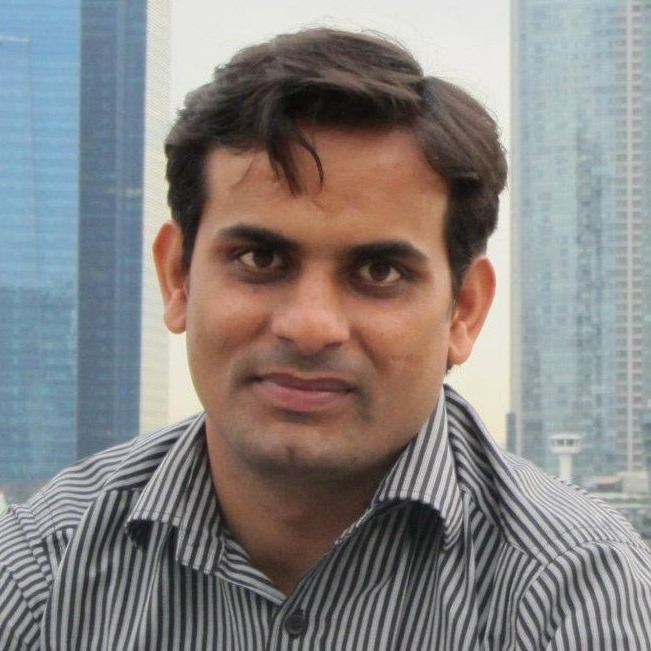Sanjeev Kumar
Professor
sanjeev.kumar[at]ma.iitr.ac.in
M-403, Mathematics Department Building, IIT Roorkee
01332-285824
MATHEMATICAL IMAGE PROCESSING (Computational Algorithms for Image Restoration; Image Encryption and Secret Sharing; Quantum Imaging; 3-D Reconstruction), MACHINE LEARNING (Neural Networks and Tree-based Classifiers; Applications in Image Processing)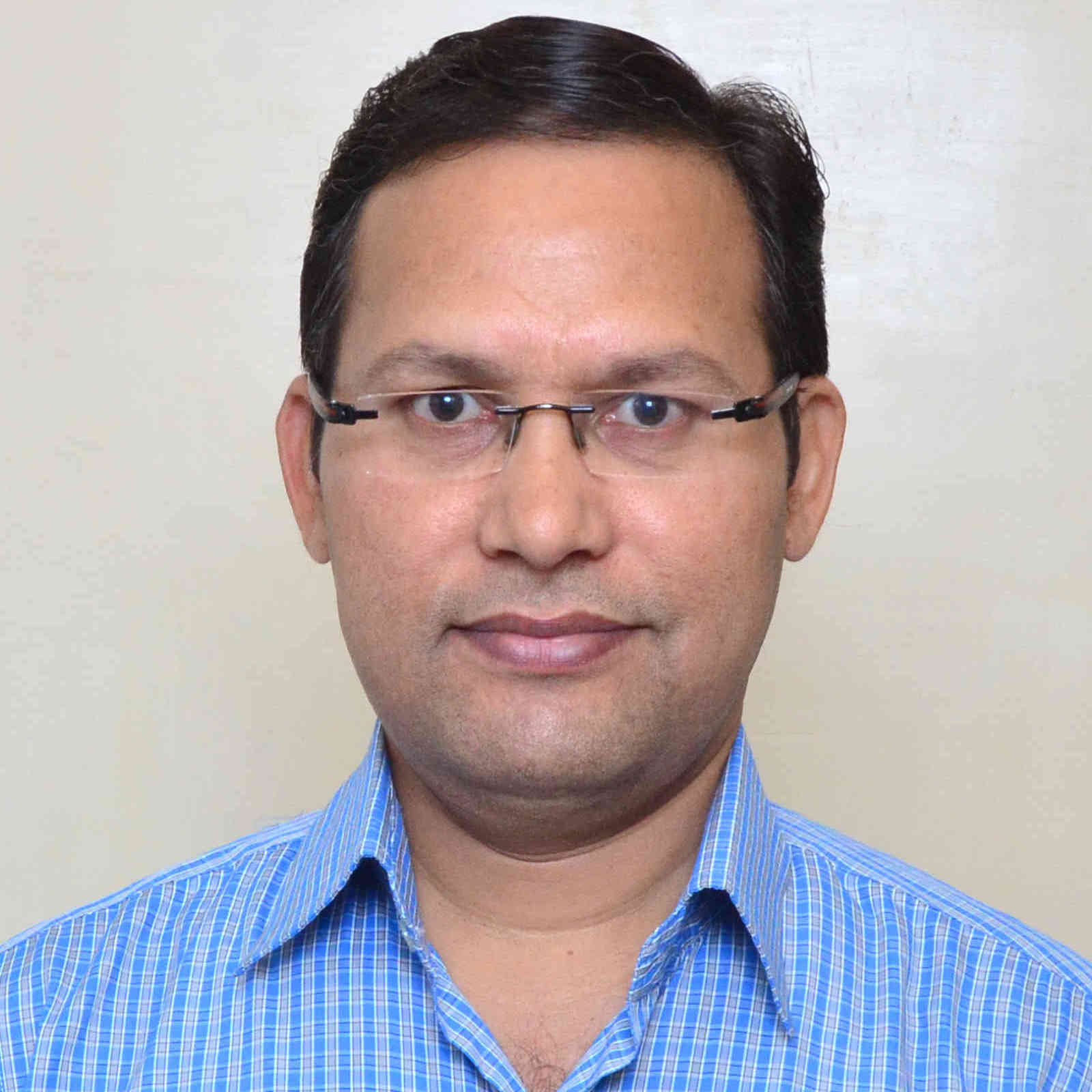Maheshanand
Professor
maheshanand[at]ma.iitr.ac.in
01332-285543
Coding Theory: Codes over rings, Locally recoverable codes, Boolean functions in coding theory and cryptography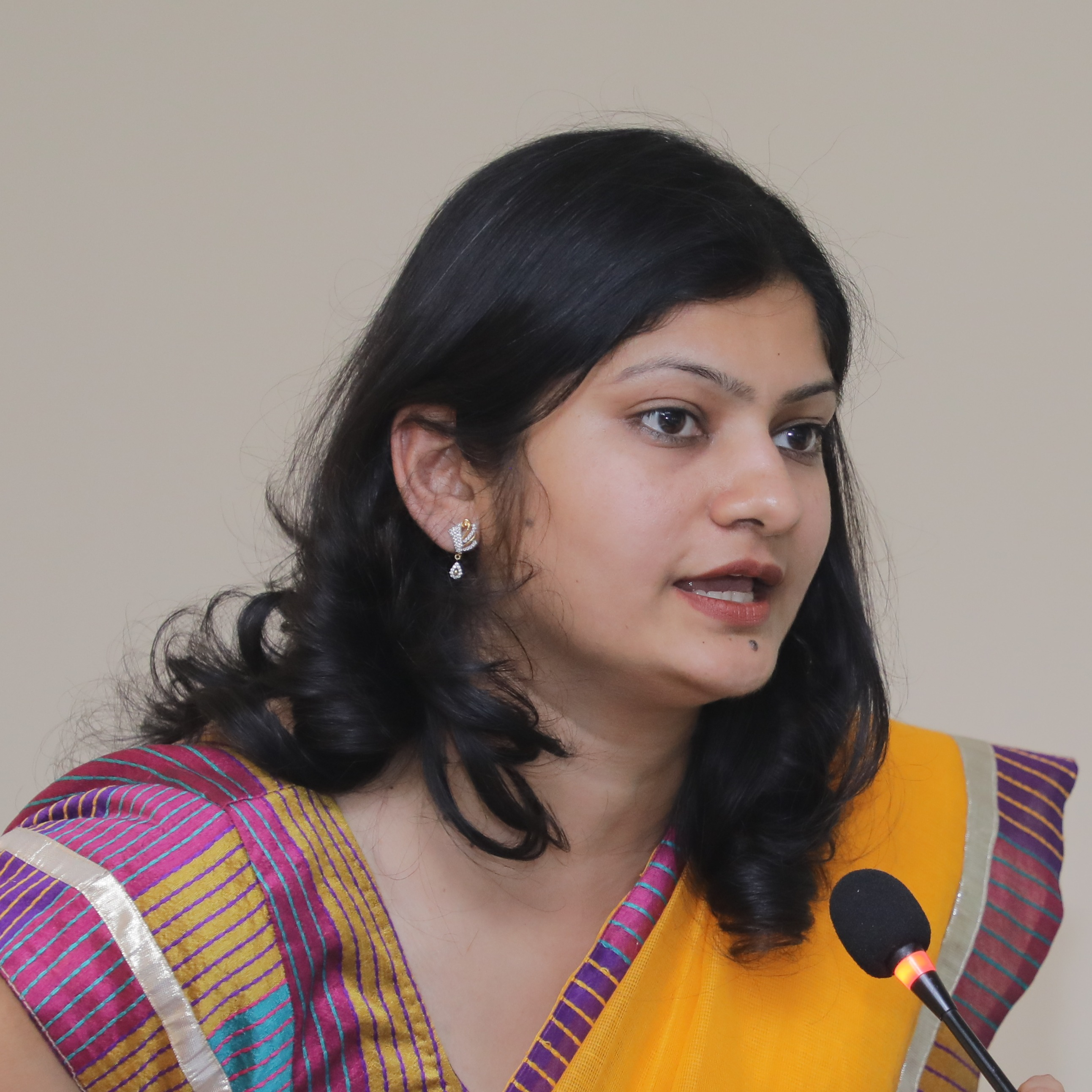Sugandha Maheshwary
Assistant professor
msugandha[at]ma.iitr.ac.in
511, Mathematics Department Building, IIT Roorkee
01332-285054
Algebra, Group rings, Unit groups, computational mathematics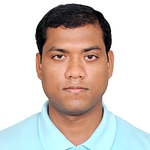Amit Maji
Assistant professor
amit.maji[at]ma.iitr.ac.in
+91-1332-285392
Operator Theory; Functional Analysis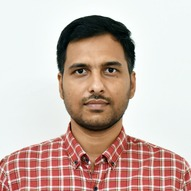Arunava Mandal
Assistant professor
arunava[at]ma.iitr.ac.in
8879261886
Lie groups, Algebraic groupsManil T. Mohan
Assistant professor
maniltmohan[at]ma.iitr.ac.in
+91-1332-285319
Stochastic (Partial) Differential Equations, Solvability, Large Deviation Theory, Ergodicity, Random Attractors, Stochastic Stability, Partial Differential Equations, Solvability and Stability of Parabolic and Hyperbolic Systems, Inverse Problems, Control Theory , Deterministic and Stochastic Control Theory in Infinite Dimensions, Optimal Control Theory, Mathematical Fluid Dynamics , Stochastic Analysis and Control of Incompressible and Compressible Fluids, Optimization, Problems with Orthogonal Matrix Constraints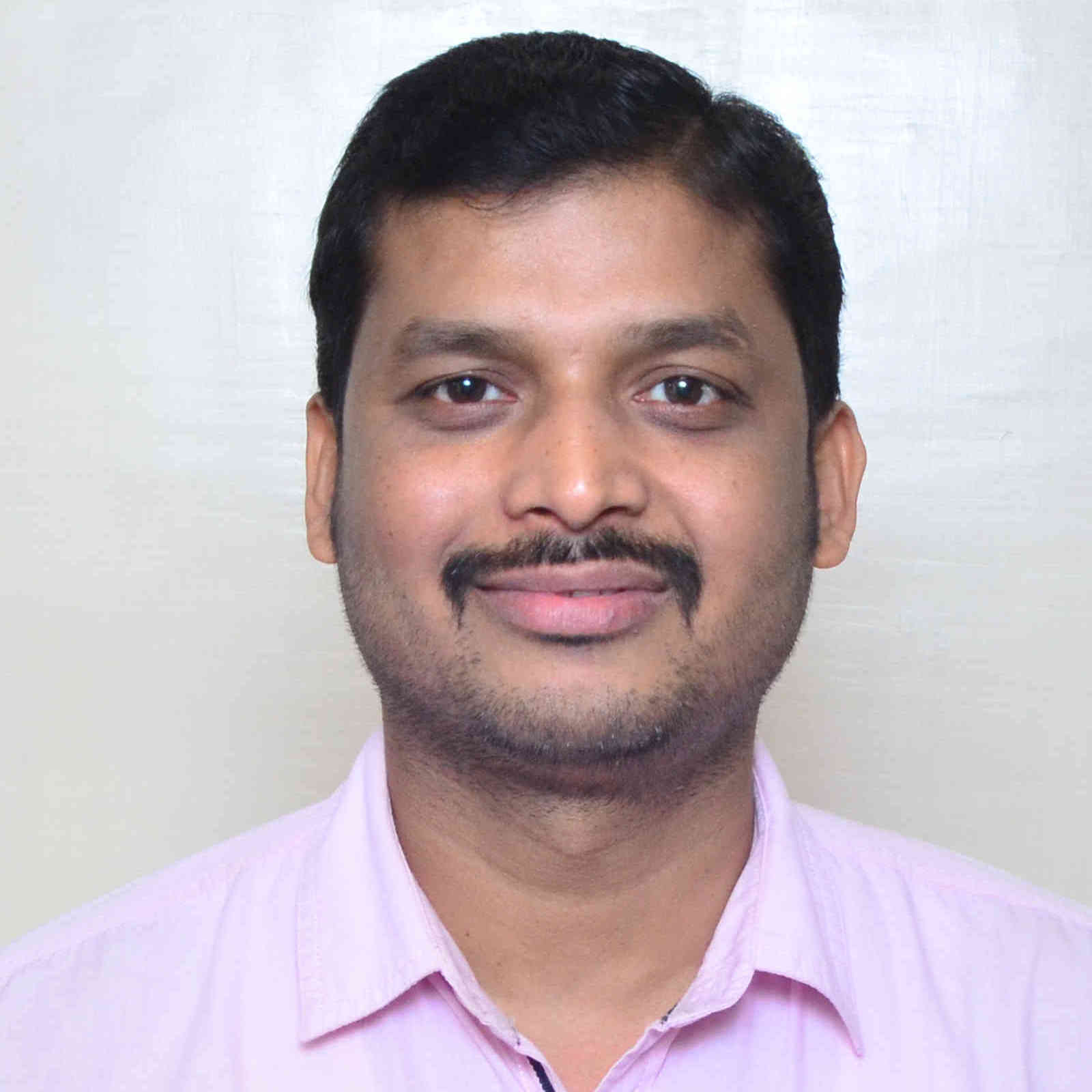Ameeya K. Nayak
Professor
ameeya.nayak[at]ma.iitr.ac.in
+91-1332-284777
Fluid Dynamics, Electroosmotic Flows, Microfluidics, Two phase flow in porous media, Ventilation problems, Computational Biophysics, Reactor Modelling, Finite element method, Population Balance Method.Ram Krishna Pandey
Associate professor
ram.pandey[at]ma.iitr.ac.in
01332-284796
Number Theory (Additive, Combinatorial, and Elementary)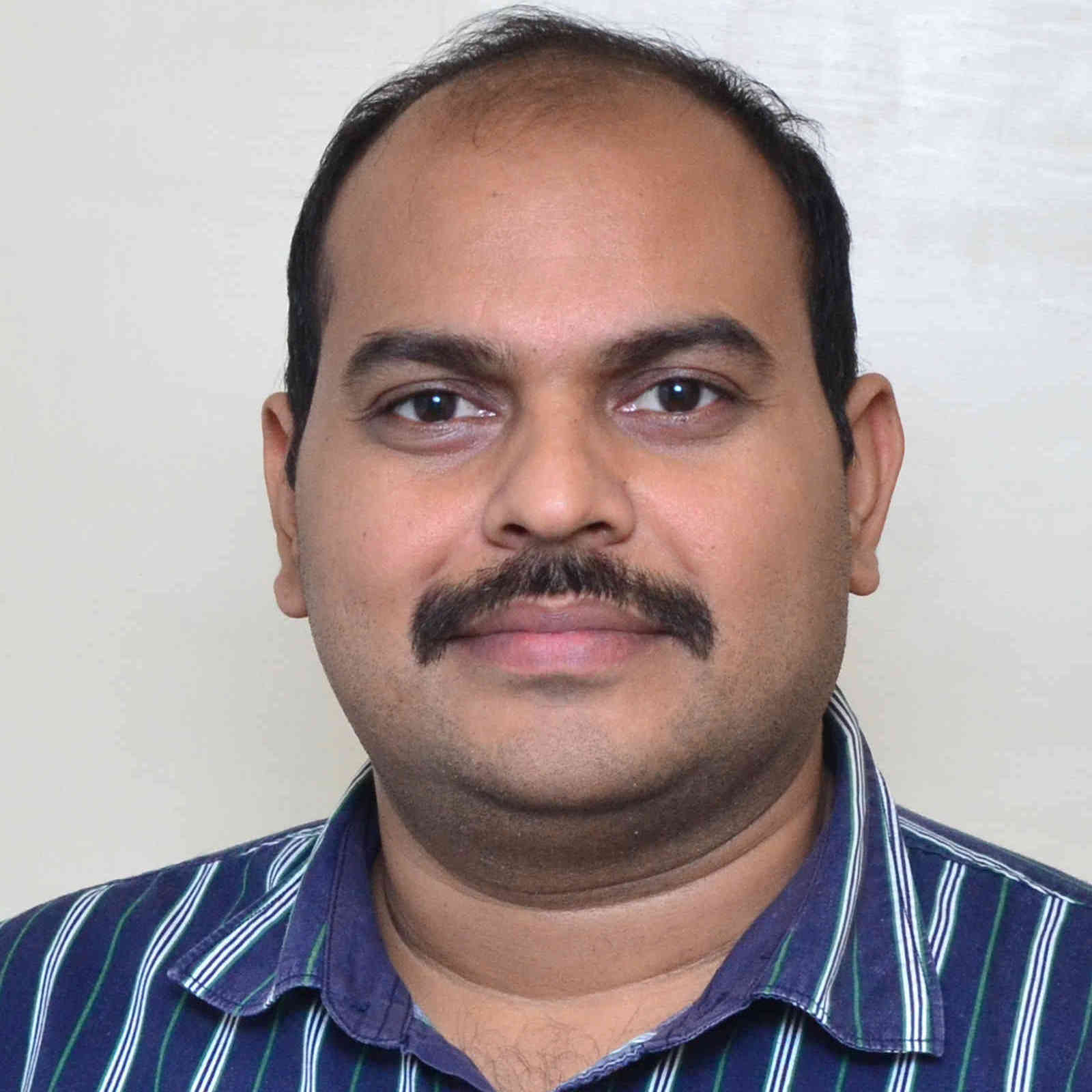D.N. Pandey
Associate professor
dwijendra.pandey[at]ma.iitr.ac.in
01332-28-5735
Differential equation, Functional Evolution Equation, Control theory, Semi-group theory, Fractional Differential EquationsPratibha
Associate professor
pratibha[at]ma.iitr.ac.in
Mob.No.+919897030710, Bio Mathematics, Computer Appl, Computational Fluid Dynamics, Lung Mechanics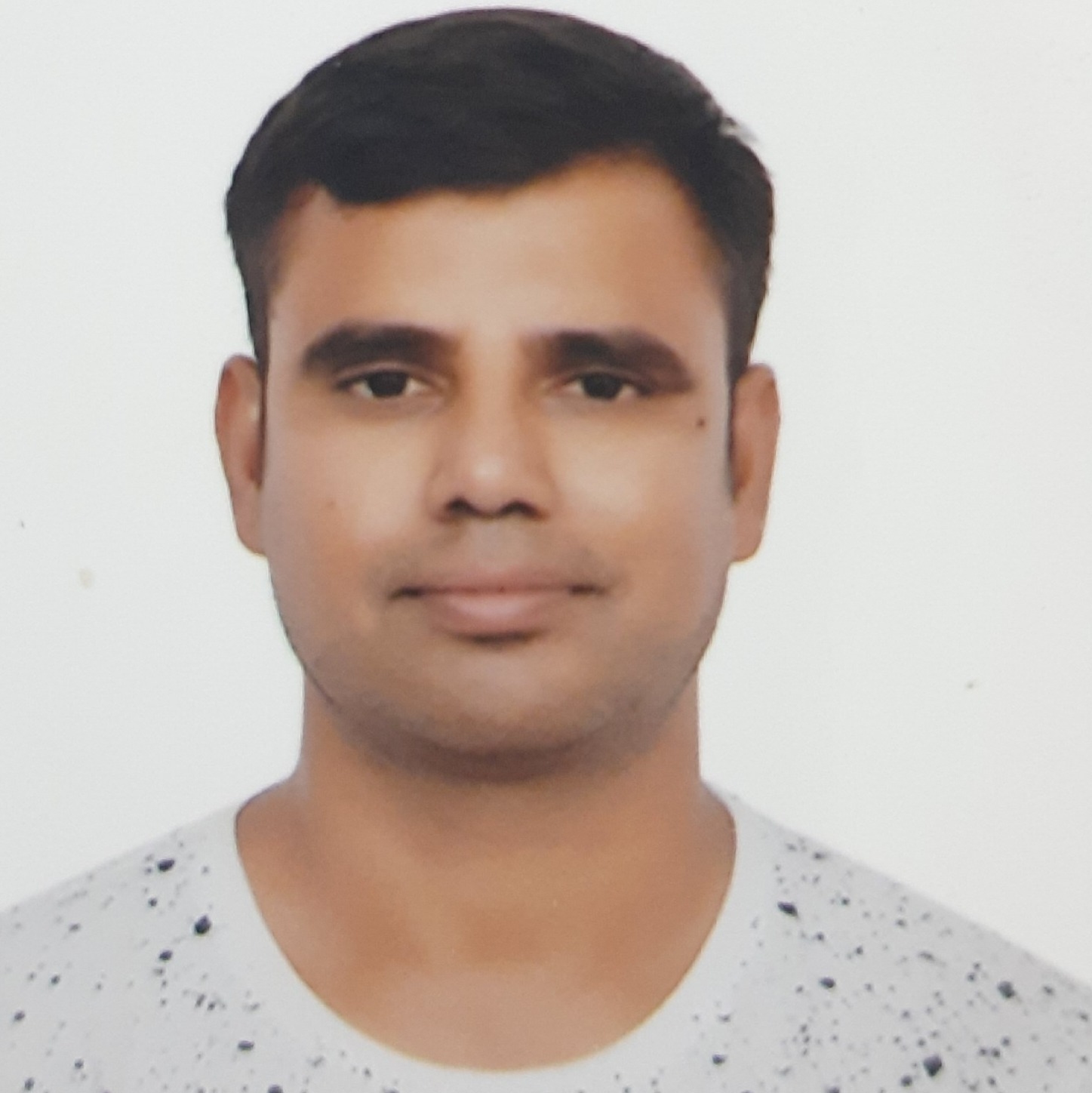Narayan Rakshit
Assistant professor
nrakshit[at]ma.iitr.ac.in
01332285209
Functional Analysis, Operator Algebras and Operator Theory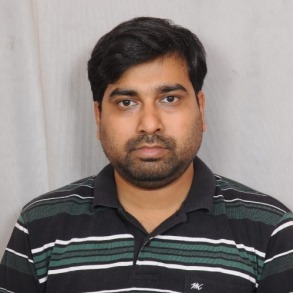Saikat Saha
Assistant professor
saikat.saha[at]ma.iitr.ac.in
9830141300
Kinetic Theory of Granular Matters and Gas-Solid Suspensions, Active Colloids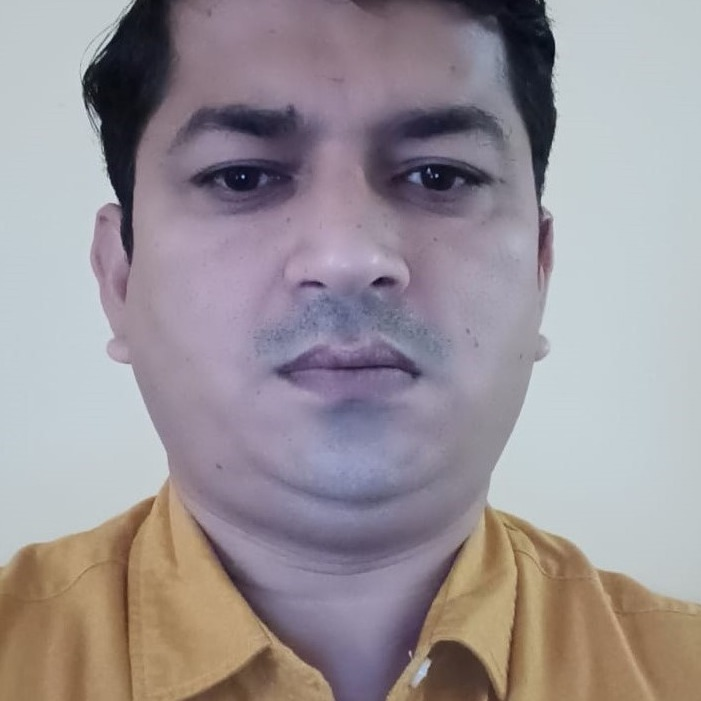Associate professor
01332285430
Approximation of functions by Fourier series, Singular Integrals, Neural Network Operators.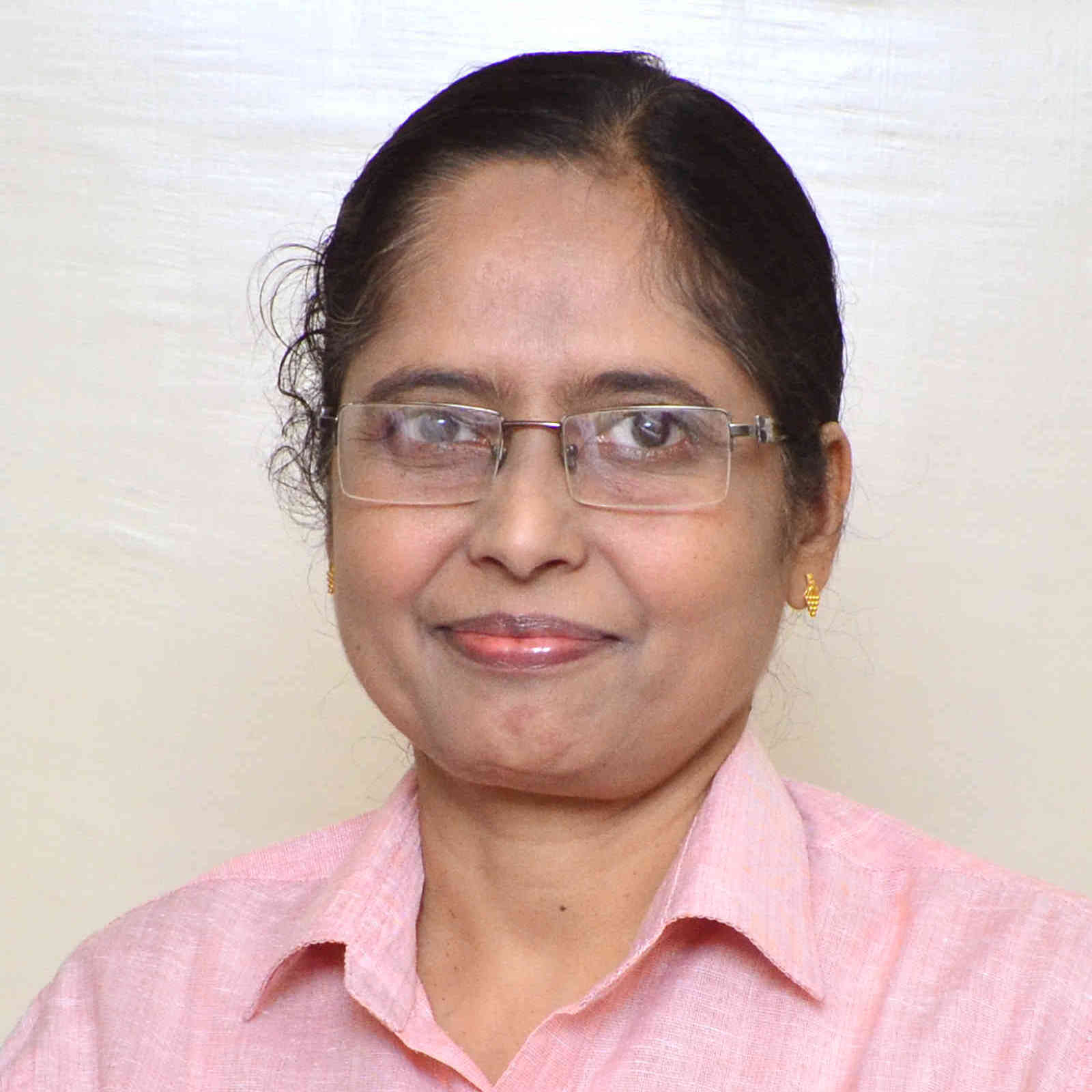Tanuja Srivastava
Professor
tanuja.srivastava[at]ma.iitr.ac.in
01332-285084
Computerized Tomography, Reconstruction Error Analysis, Convolution Backprojection, Image Processing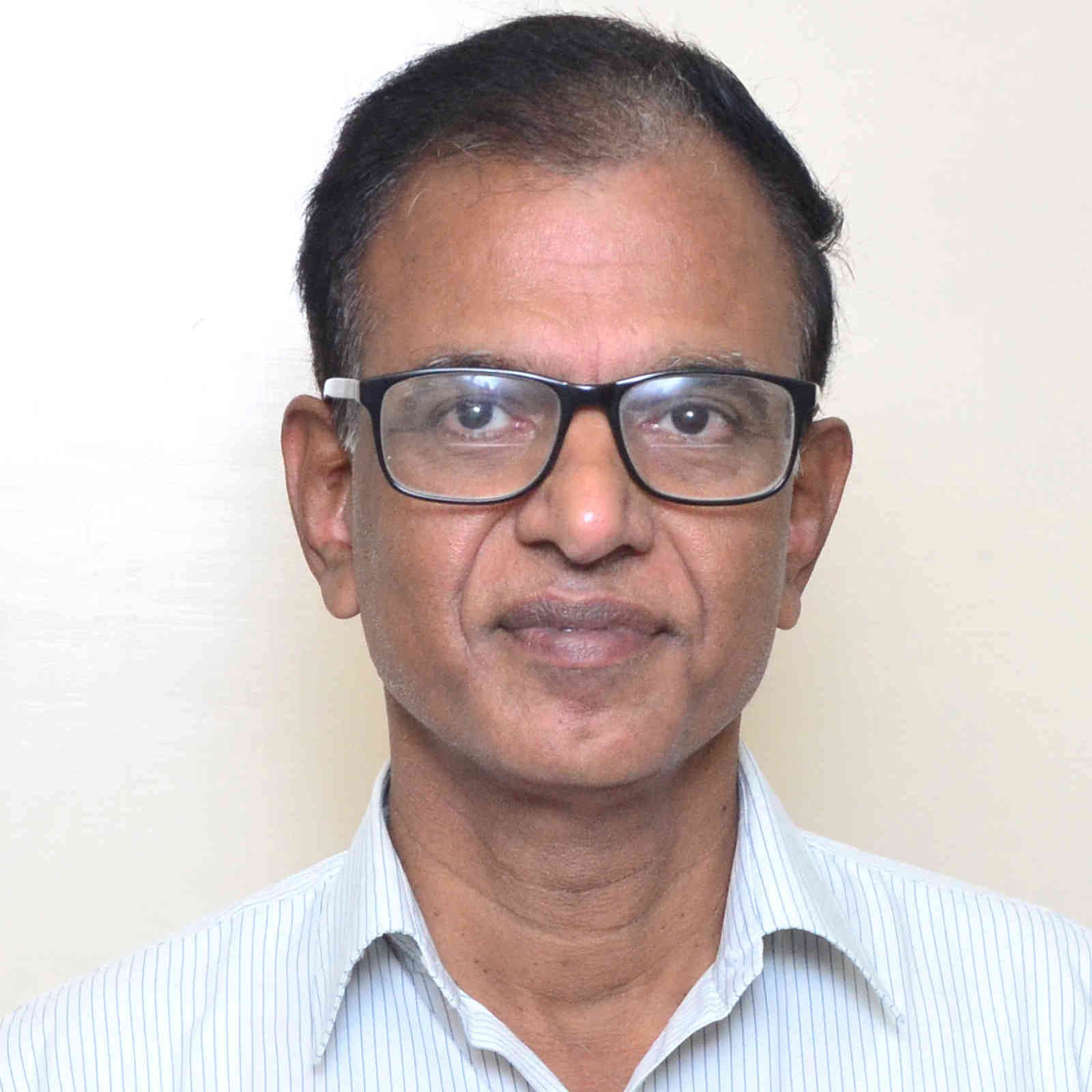N. Sukavanam
Professor
n.sukavanam[at]ma.iitr.ac.in
+911332285341
Nonlinear Analysis, Solvability of operator equations, Inverse problems, Control Theory, Controllability, stability and stabilizability, Fractional order systems, Robotics and Control, Trajectory tracking controller, Biped Robot Control, ANN based controlA. Swaminathan
Professor
a.swaminathan[at]ma.iitr.ac.in
01332-285182
Complex Analysis, Orthogonal Polynomials and Special Functions, Functional Analysis: Operator Theory and Approximation Theory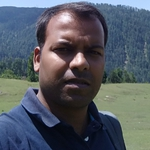Mahendra Kumar Verma
Assistant professor
mahendraverma[at]ma.iitr.ac.in
9769586280
Number Theory, Automorphic representations
Retired Faculty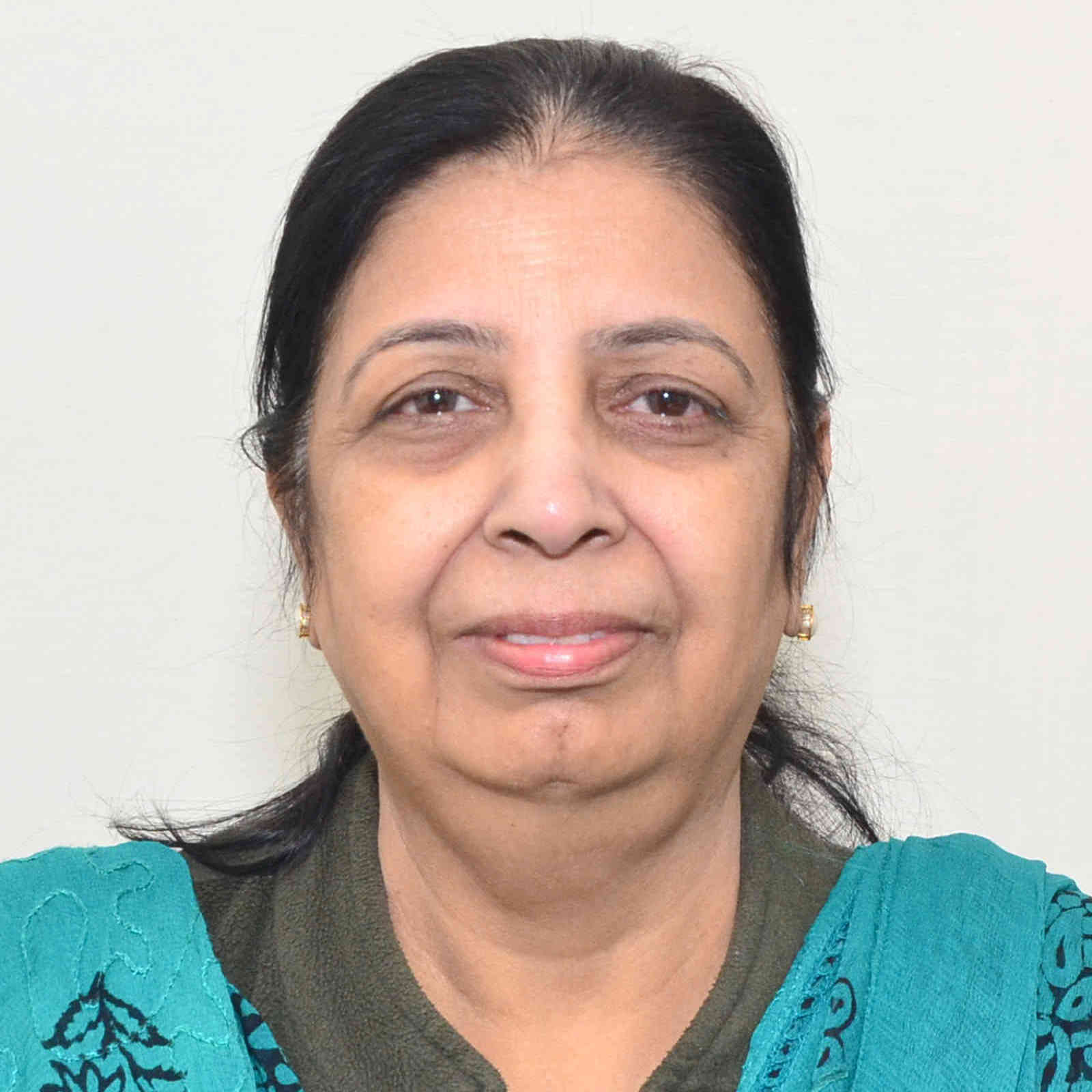Sunita Gakkhar
Retired faculty
sungkfma[at]iitr.ac.in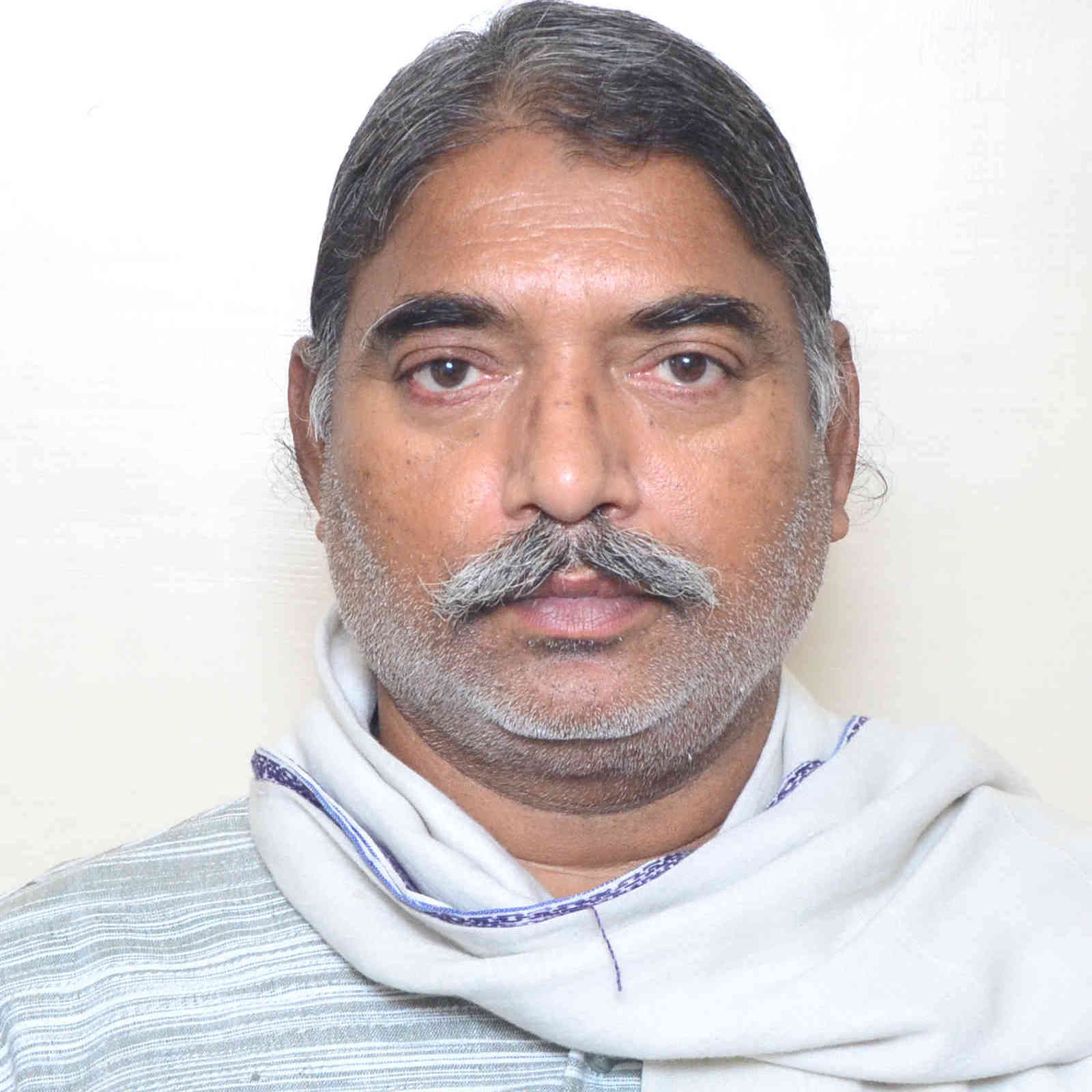Retired faculty
+91-9458121637
Operations Research, Optimal Control Theory, Fuzzy Maths, Intuitionistic Fuzzy Maths, Data Envelopement Analysis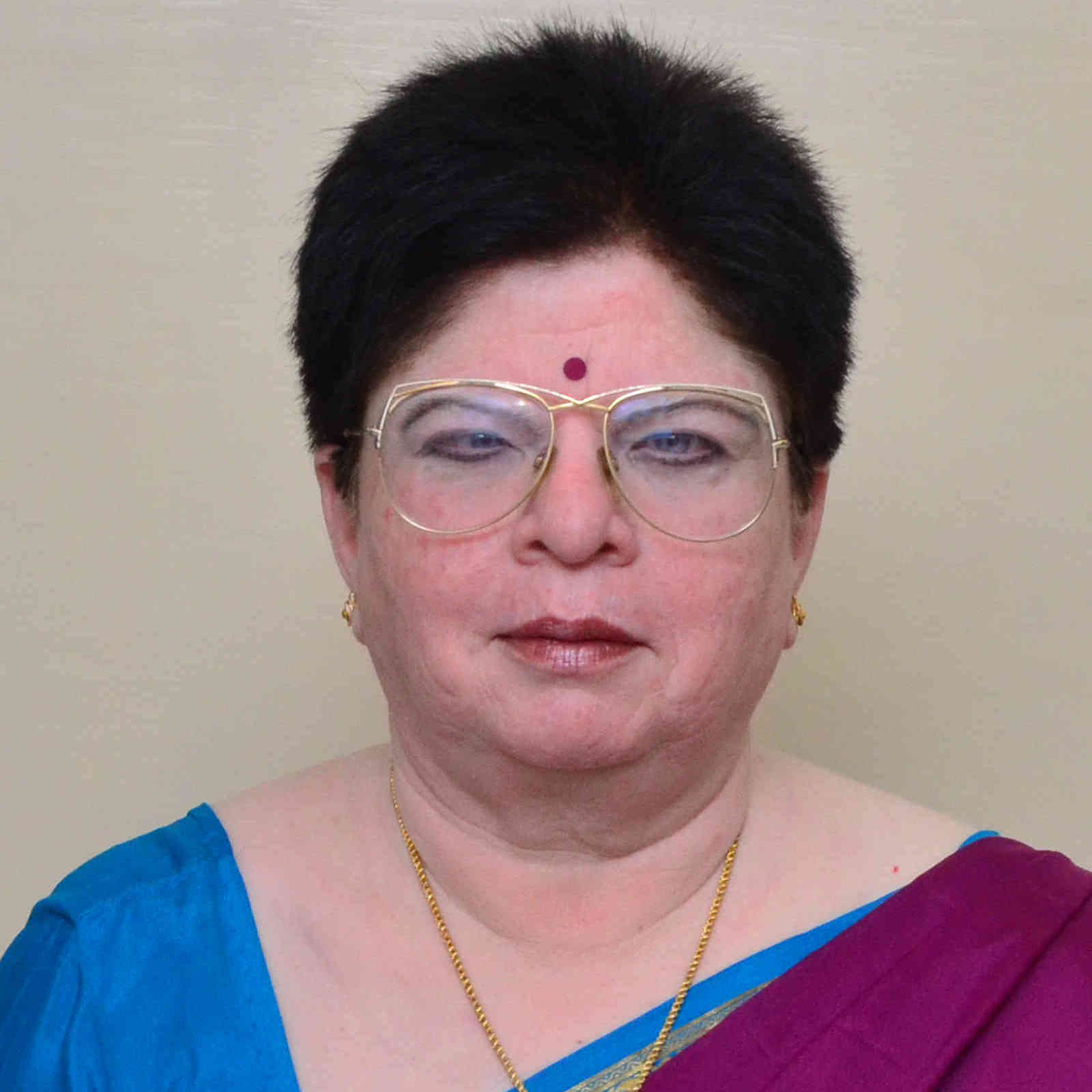Kusum Deep
Retired faculty
kusum.deep[at]ma.iitr.ac.in
First Floor, New Building of Department of Mathematics
09837164078
Operations Research, Nature Inspired Optimization Techniques, Soft Computing, Evolutionary Algorithms, Swarm Intelligence Techniques, Artificial Intelligence and Data Science, Parallel ComputingR.R. Bhargava
Retired faculty
rajrbfma[at]iitr.ac.in
Applied Mathematics,Mathematcal Modelling, Smart Material Fracture, Piezoecltricity, Elasticity,Frac, Elasticity,fracture Mechanics Theory,numerical Analysis , Mathematcal Modelling,Applied Maths(Solid Mechanics), Smart Material Fracture, Piezoecltricity, ElasT.R. Gulati
Retired faculty
trgorfma[at]iitr.ac.in
Operations Research (Mathematical Programming) , Operations Research, Mathematical Programming and Duality Theory.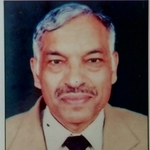M.L. Mittal
Retired faculty
mymitfma[at]iitr.ac.in
01332285313
Mathematical Analysis, Fourier Analysis, Approximation Theory, Summability Theory, Functional Analysis, Numerical Analysis